# Excel

Excel is a computer software program by Microsoft that is used for storing, organising and manipulating data.

It can be used for simple tasks such as calculating sums and percentages, and create graphs and charts to present your data. You can also use it for statistical analysis including finding means and standard deviation. Complex analysis is also possible with Excel, including analysis of variance (ANOVA) and correlations.

Check in with our IT service to download free updates to Office 2016 and other programs. We recommend you keep your software up-to-date for security and usability purposes.

If you are new to Excel, watch this 30-minute video. It gives an overview of using the different functions of Excel.

#### Entering a formula in Excel

You can enter a formula to calculate things such as sums, percentages, averages, standard deviations and more.

For Excel to recognise a formula, begin with ‘=’. For example, if you type ‘=2+3’ in a cell followed by enter, excel will calculate this for you to be five. The same method can be used for adding values in cell. For example, typing =(A2+A5) and then enter, will add the contents in cell A2 with the contents in cell B5. If you wanted the result of this formula to appear in cell A7, you would do the following:

1. Select cell A7
2. Type =
3. Select cell A2
4. Type +
5. Select cell A5
6. Press Enter

You could also select cell A7 and type the full formula =A2+A5 followed by enter.

Mathematical operations

The following symbols are used in excel for mathematical operations:

 Symbol Mathematical operation + (plus sign) Addition – (minus sign or hyphen) Subtraction * (asterisk) Multiplication / (slash) Division ^ (caret) Raising a number to an exponential power

There are several more advanced options in Excel. Find all mathematical operations under the tab ‘Formulas’ (see Figure 1).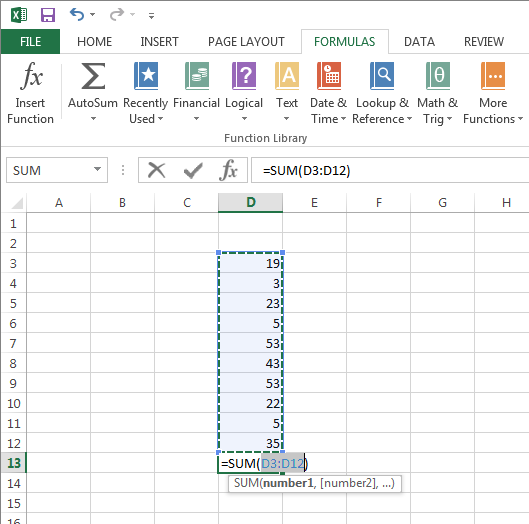Figure 1: Formulas in Excel

For example, to find the sum of the content of a range of cells, highlight the relevant cells and then click ‘AutoSum’. The formula for sum will immediately appear in the cell below the highlighted cells (Figure 1).

For more support, please refer to the following pages:

Creating a formula

How to fix a broken formula

#### Statistical analysis using Excel

To perform statistical analysis in Excel, you need an add-in called Analysis ToolPak. How to install the Excel ToolPak.

Excel can be used to perform various different statistical analyses. For example, by clicking ‘More functions, Statistical, AVERAGE’ you can calculate the average (mean) of any cells.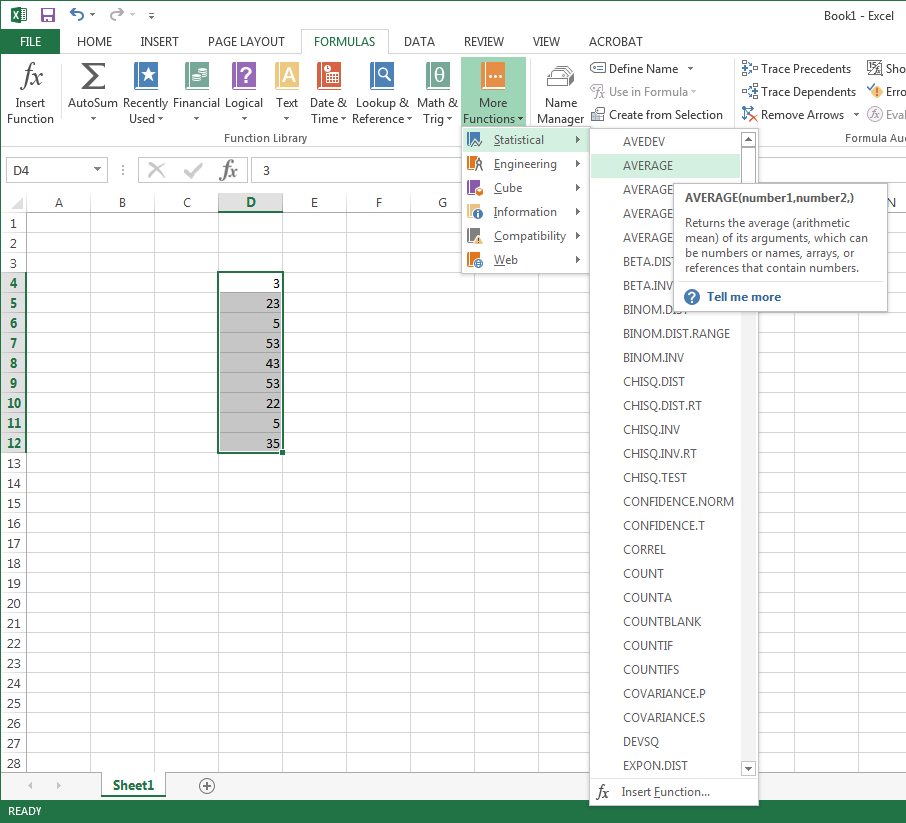Excel will then tell you to specify what cells you want to average. You can either type these in manually (or if it is a range of cells, you can simply write the first cell and the last cell, separated by a colon).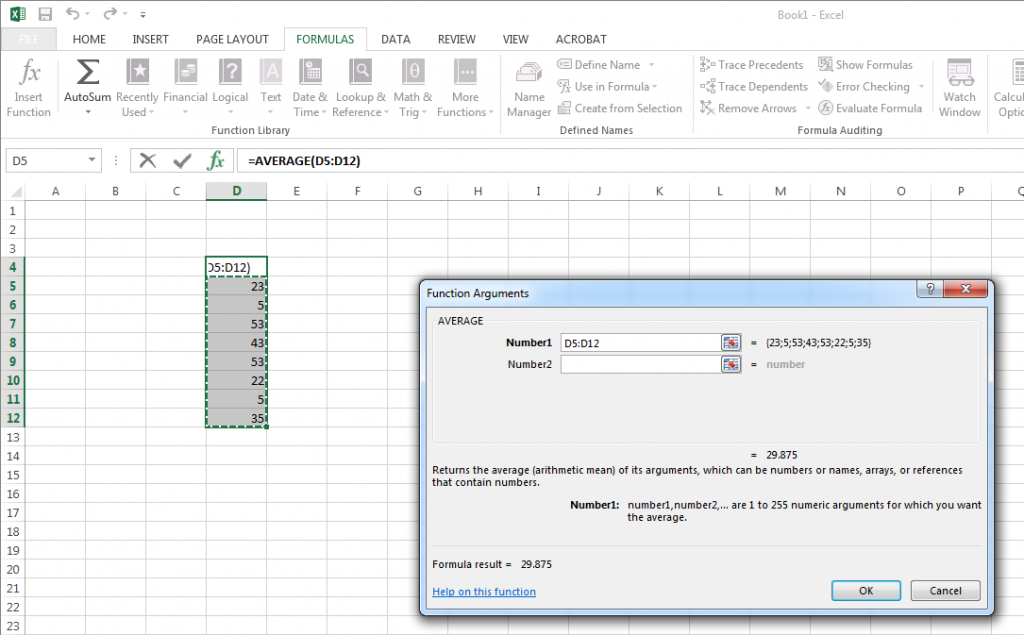Read more about the statistical analysis functions available in Excel and instructions on how to use them.

#### Making a graph or chart

Excel is a tool for creating graphs to present your data. You can create many different types of graphs, including bar graphs, pie charts, line graphs, area graphs and radar graphs.

To create a graph, first select your data. You can highlight two different sections by pressing Ctrl.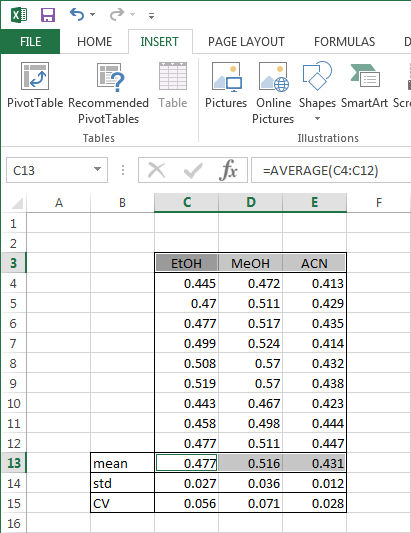In this example, we are creating a bar graph that will illustrate the difference between the means of three different solutions.

1. Highlight the mean data values
2. Press and hold Ctrl, then
3. Highlight the data labels.

Note 1: These are the labels that will pop up in your graph, so make sure they are in the format you want.

Once this is done, go to the ‘Insert’ tab, where you will find a number of different graphical options. For a bar graph, select the bar graph icon.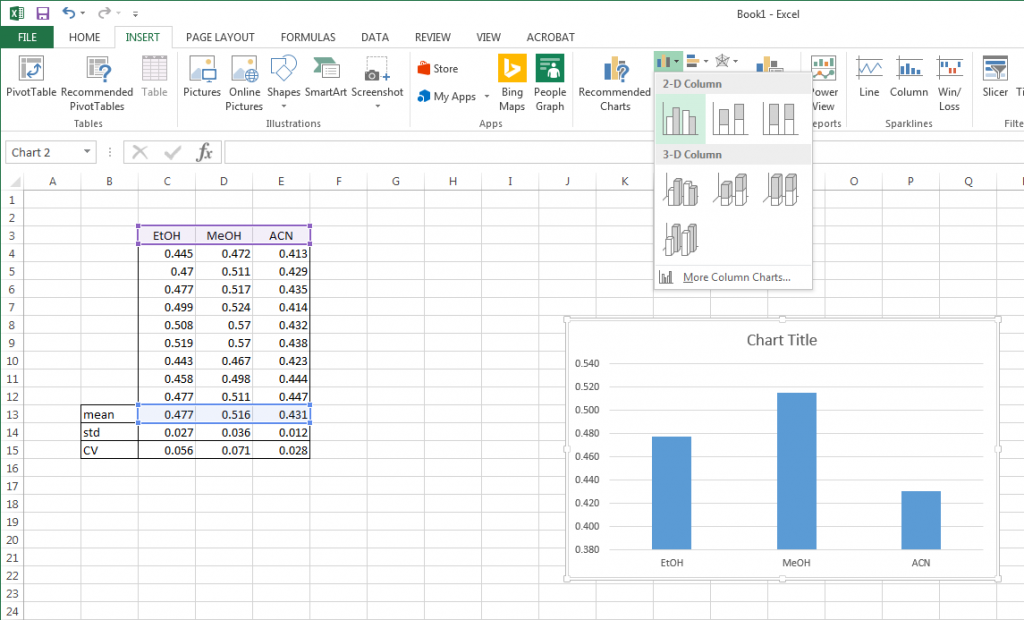If the graph does not look as you expected, click on the ‘switch row/column’ button, and the axes will be reversed.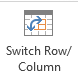Next right-click (Ctrl click for Apple users) on the chart and select Move Chart. By doing this, you can move the chart into its own tab.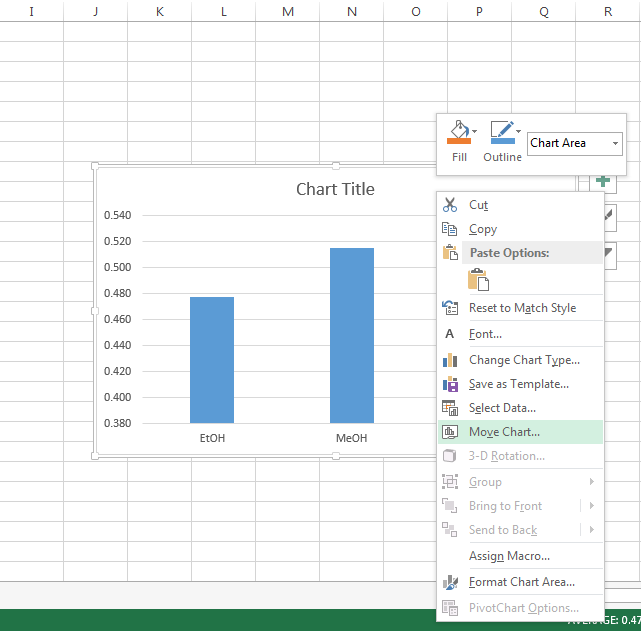As you click on the ‘Move Chart’ above, select ‘New sheet’ and give it a name. Click OK.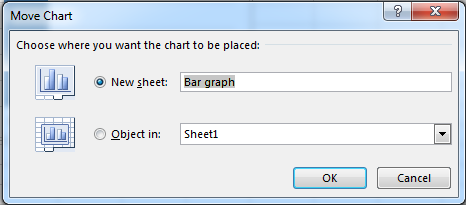Your graph will now appear in a full-screen format, making it easier for you to edit.

Learn about chart elements and how to edit the visual aspects of your chart.